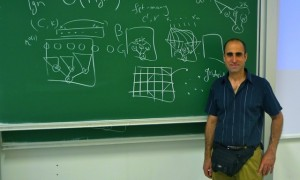# Iyad Kanj

## What makes normalized weighted satisfiability tractable

VCLA hosted a talk by Iyad Kanj on June 22nd, 2012.

 DATE: Monday, June 25, 2012 TIME: 11:00 c.t. VENUE: Seminarroom Goedel (Favoritenstrasse 9-11, ground floor, access through courtyard)ABSTRACT

We consider the weighted antimonotone and the weighted monotone satisfiability problems on normalized circuits of depth at most t >= 2, abbreviated wsat^-[t] and wsat\$^+[t], respectively. These problems model the weighted satisfiability of antimonotone and monotone propositional formulas (including weighted antimonotone/monotone cnf-sat) in a natural way, and serve as the canonical problems in the definition of the parameterized complexity hierarchy. We characterize the parameterized complexity of wsat^-[t] and wsat^+[t] with respect to the genus of the circuit. For wsat\$^-[t], which is W[t]-complete for odd t and W[t-1]-complete for even t, the characterization is precise: We show that wsat^-[t] is fixed-parameter tractable (FPT) if the genus of the circuit is n^{o(1)}, where n is the number of the variables in the circuit, and that it has the same W-hardness as the general wsat^-[t] problem (i.e., with no restriction on the genus) if the genus is n^{Omega(1)}. For wsat^+ (i.e., weighted monotone cnf-sat), which is W-complete, the characterization is also precise: We show that wsat^+} is FPT if the genus is n^o(1) and W-complete if the genus is n^{Omega(1)}. For wsat^+[t] where t > 2, which is W[t]-complete for even t and W[t-1]-complete for odd t, we show that it is FPT if the genus is O(sqrt{log{n}}), and that it has the same W-hardness as the general wsat^+[t]} problem if the genus is n^{Omega(1)}.

Comments are closed.